# Math Practice Topic: Pythagorean Theorem

 Description: Find the missing sides of a triangle using the Pythagorean Theorem. Adaptive Learning Progression: Numbers get bigger. Start using MathScore for free

## Sample Levels (out of 4)

Find the value of 'x' using the Pythagorean Theorem.

 1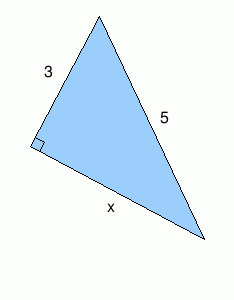x = 2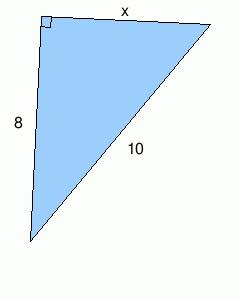x = 3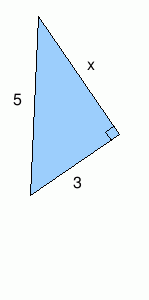x =

Find the value of 'x' using the Pythagorean Theorem.

 1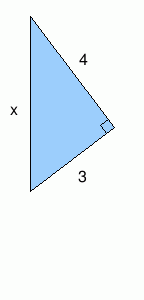x = 2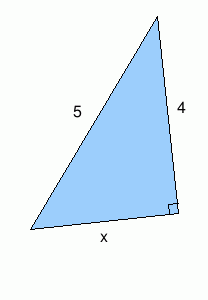x = 3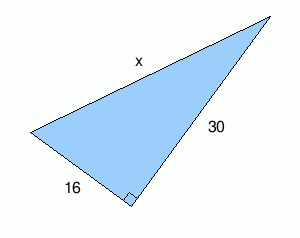x = 4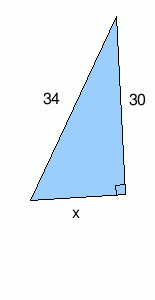x = 5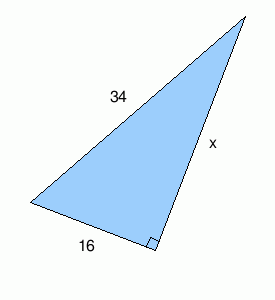x =